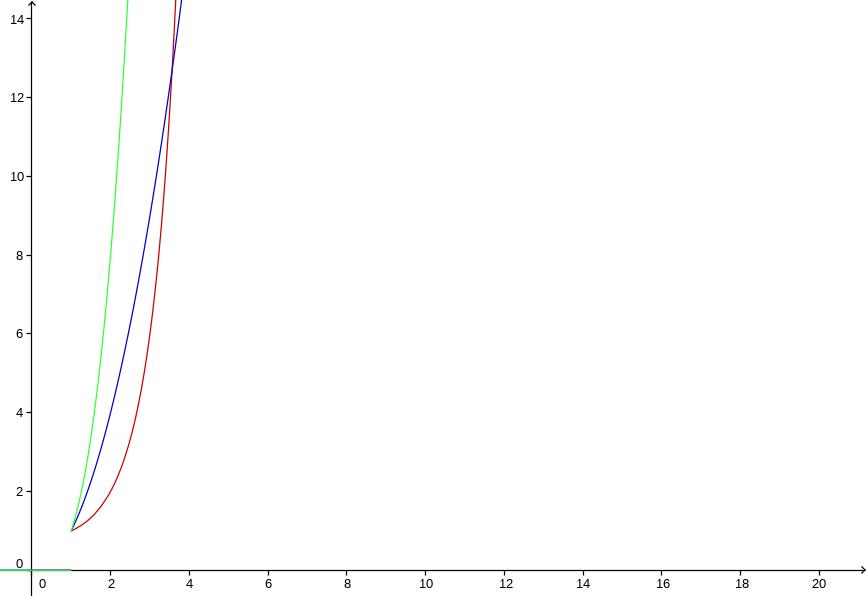# 排列与组合

## 阶乘

$$n! = \prod^n_{i=1} i \tag{1.1}$$## 排列

$$a, b, c$$

2排列为：
$$ab, ac, ba, bc, ca, cb$$

3排列为：
$$abc, acb, bac, bca, cab, cba$$

$$P(n, r) = {n! \over (n-r)!} \tag{2.1}$$

$$P(n, r) = \prod^n_{i=n-r+1} i = {n! \over (n-r)!}$$

$$\{a,b,c\}, \{a,b,d\}, \{a,c,d\}, \{b,c,d\}$$

$${n \choose r}r! = P(n,r) \tag{3.1}$$

$${n \choose r} = {n! \over (n-r)!r! } \tag{3.2}$$

## 组合数的基本性质

$${n \choose r} = {n \choose n-r} \tag{4.1}$$

$$\sum^n_{i=0} {n\choose i} = 2^n \tag{4.2}$$

1. 当然也可记作$_nP_r$$P^n_r。 2. 《组合数学》中称之为”子集”，也许更为恰当。 3. 下面将使用集合的方式表示组合，如\{a,b,c\}。 4. 也可记作_nC_r$$C(n,r)$$C^n_r$。